home | publications | research | links | contactresearch networks Networks are the embodiment of interacting particles (nodes representing people or emotions, variables) to generically represent complex systems. Because they are simplifications of real systems, they allow for rigorous analysis and simulations. Such analyses may reveal bistable patterns or correlations with empirical findings. They also allow for a more mechanistic explanation of phenomena. We also consider mixed graphical models with discrete and continuous random variables. [P28,P33,P49,P61,P76,P94]causality Causal relations are central in all disciplines of science. The modern view of causality is that of an intervention: If $X\to Y$ is a causal relation, then if $X$ is changed then $Y$ is changed as a consequence. This seems to be a working definition (although not made very precise here). Discovering causal relations is becoming more commmon in psychology. It is possible to combine different dataseets from observational and experimental (i.e., interventional) settings, to determine more accurately the causal relations. [P81]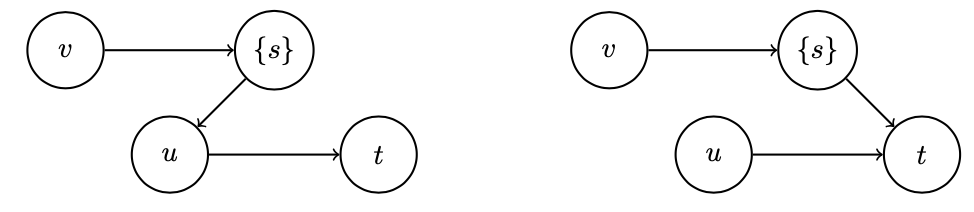dynamical systems Dynamics are often described by differential equations (ordinary, partial, stochastic). These describe development of the states over time. We investigate several types of dynamical systems, including those connected to disease spreading, where we use these to repreesent the evolution of symptoms of disorders. [P43,P75,P92]cellular automata An interesting mix of networks and dynamical systems is the field of cellular automata. A cellular automaton is a network (often with nodes that are 0 or 1) with a certain topology connecting the nodes where the 0-1 pattern changes over time. The changes are brought about by the influence of connected nodes (neighbours). We have investigated mean field approximations of stochastic cellular automata which may aid explanations of psychpathology onset. [P75,P93]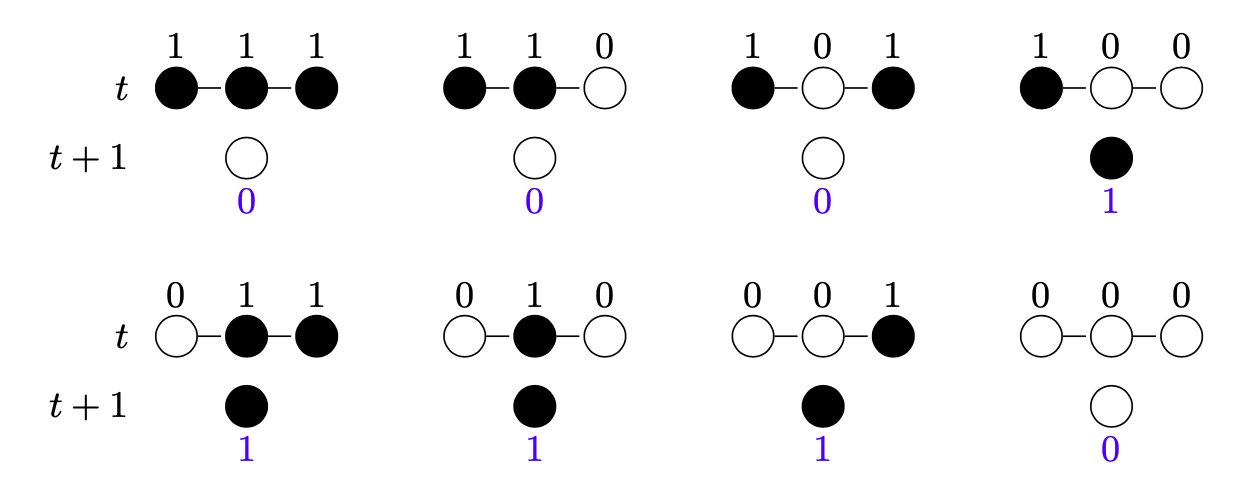psychometrics in networks Psychometrics deals with the foundations of psychological models and their interpretation, encompassing measurement and modeling. We have developed several methods to base standard psychometric interpretations of test results on networks. One way is to consider a latent variable as superfluous and deal directly with network of responses (e.g., the Ising model). Another approach is to consider what properties a decision function (e.g., pass/fail) should have which can be decomposed using Fourier analaysis of Boolean functions. [P61,P65,P72,P95]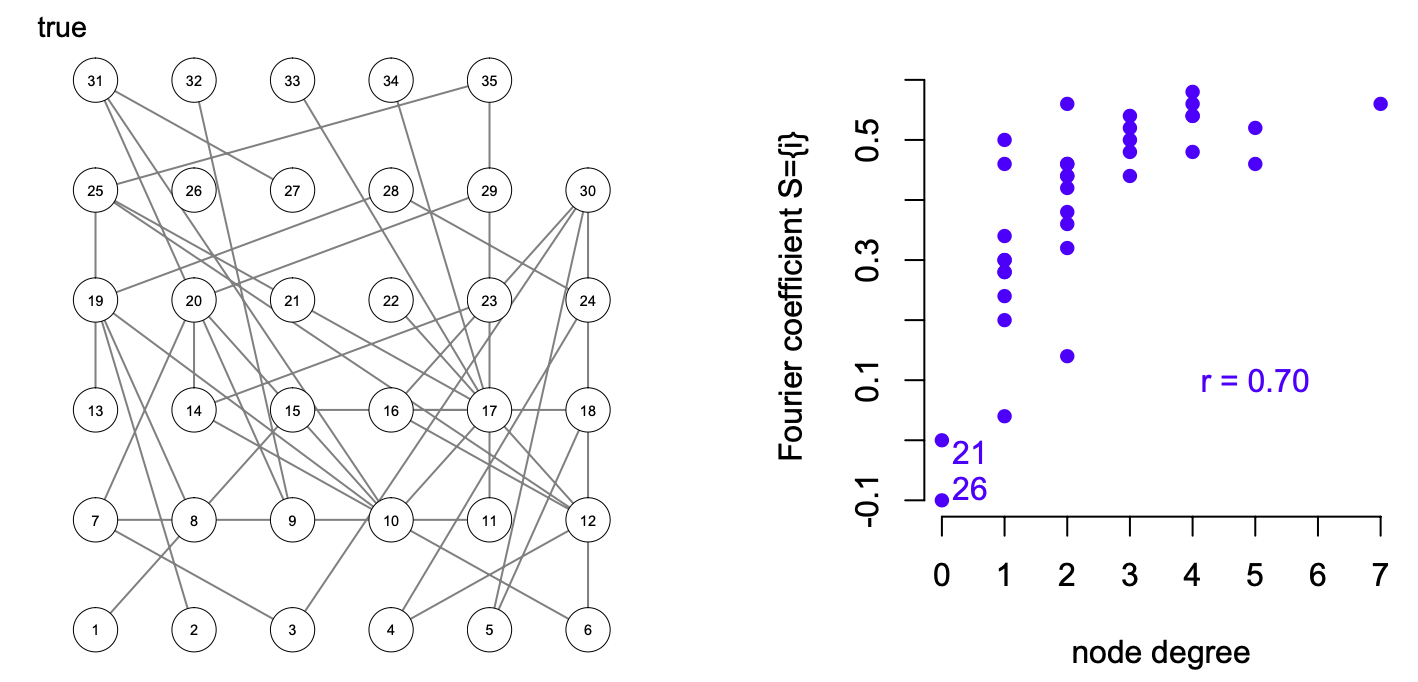randomness and foundations of probability Defining probability is not as easy as you might think. First, try to define randomness. Von Mises tried to define it by two axioms (exisitence of limit and admissible place selection of the limit). This axiomatisation turned out to be inadequate, for instance, it does not satisfy the theorem of the itereated logarithm (a strong law of large numbers). Probability is founded upon these notions. What is the best way to move forward? [Notes on probability and statistics] regularization techniques The possibility of gathering large amounts of data, especially in the number of variables, has become increasingly popular. This has led many to study properties of estimation using certain restrictions on the type of obtained solution. The most well-known examples are the lasso and ridge estimators. Violation of the assumptions may lead to rather counterintuitive situations, like predicting well but poor recovery. [P68]model selection In many types of research the objective is to obtain an accurate representation of a data generating system. For instance, a set of coupled differential equations representing the development of mental abilities. Estimates are used to obtain information on the parameters involved, but to have some idea of whether the model is a good representation, model selection is required. Model selection can also be described by separating noise from signal (in additiva and multiplicative models). Many ideas have been proposed and it seems that there is no single solution to all situations. [P25]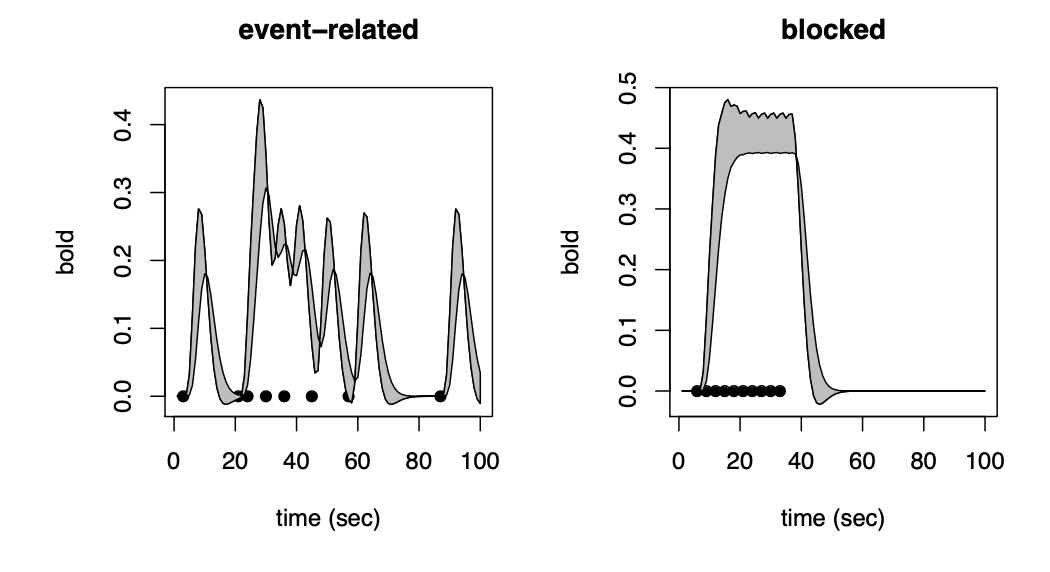optimisation (convex) and linear programming Formalising and implementing quantifiable problems has resolved many long standing issues. For instance, the train schedule has been tackled by considering the constraints of riding on time and availability of personnel and many other constraints, optimising travel time. If the problem can be described by a linear equation, then a linear program can be used for optimisation. In network theory, assortative mixing (degree correlation) can better be described by the so-called s-metric. This metric can be represented by a linear program and optimisation of the highest s-metric graph can be obtained. [P56]applications of networks Most of the applications of networks involve psychopathology. In applications we focus on determining the fit of a model to the empirical data. Evidence of bifurcations obtained from a mean field for stochastic cellular automata, for instance, is found in patiients. [P25]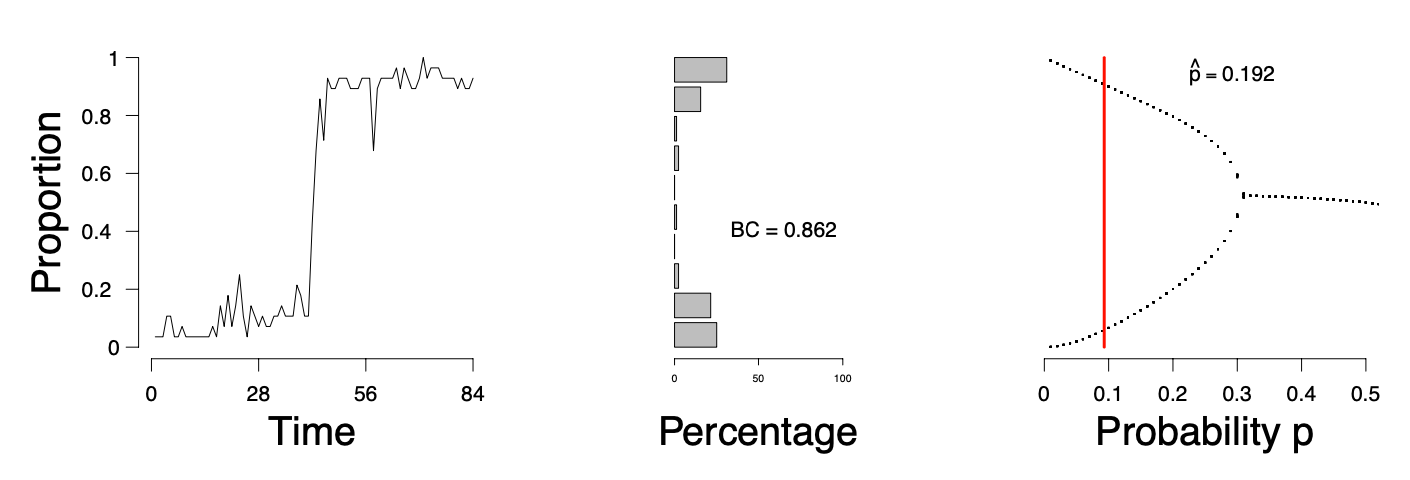asymptotic statistics In the realm of nice statistical results, like the t-test, all is well when we have a suffucient number of observations. However, often we need to know how close we are to this ideal situation, where the empirical distribution function is close enough to a normal distribution, for instance. Results like the Berry-Esseen or Glivenko-Cantelli theorems tell provide us with a bound on the error we are making given a fixed number of observations. [P76, P92]